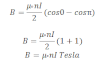# Magnetic Field Along Axis Of Solenoid

A solenoid is a ling cylindrical coil having number of circular turns. Consider a solenoid having radius R consists of n number of turns per unit length. Let P be the point at a distance x̥ from the origin of the solenoid where we have to calculate the magnitude of the magnetic field. The current carrying element dx at a distance x from origin and a distance r from point P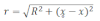The magnetic field due to current carrying circular coil at any axis is: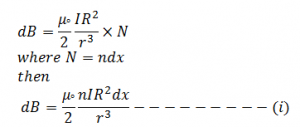From figure we have,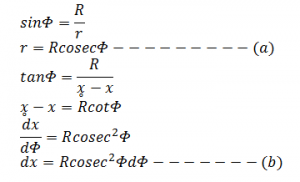Now from above three equations, we get,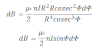Now total magnetic field can be obtained by integrating from Φ1 to Φ2, we get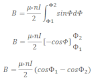Hence this expression gives the magnetic field at point p of the solenoid of finite length.For infinite long solenoid

so,